Search

Synthetic Division & Factoring (page 4 of 4)

Sections: Introduction, Worked examples, Finding zeroes, Factoring polynomials• Use synthetic division to determine whether x  4 is a factor of:

2x5 + 6x4 + 10x3  6x2  9x + 4

• For x  4 to be a factor, you must have x = 4 as a zero. Using this information, I'll do the synthetic division with x = 4 as the test zero on the left: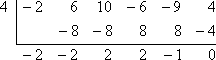Since the remainder is zero, then x = 4 is indeed a zero of 2x5 + 6x4 + 10x3  6x2  9x + 4, so:

Yes, x  4 is a factor of 2x5 + 6x4 + 10x3  6x2  9x + 4

• Find all the factors of 15x4 + x3  52x2 + 20x + 16 by using synthetic division.

Remember that, if x = a is a zero, then x  a is a factor. So use the Rational Roots Test (and maybe a quick graph) to find a good value to test for a zero (x-intercept). I'll try x = 1: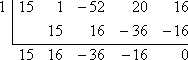This division gives a zero remainder, so x = 1 must be a zero, which means that  x  1 is a factor. Since I divided a linear factor (namely, x  1) out of the original polynomial, then my result has to be a cubic: 15x3 + 16x2  36x  16. So I need to find another zero before I can apply the Quadratic Formula. I'll try x = 2: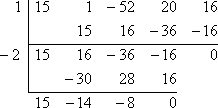Since I got a zero remainder, then x = 2 is a zero, so x + 2 is a factor. Plus, I'm now down to a quadratic, 15x2  14x  8, which happens to factor as:

(3x  4)(5x + 2)

Then the fully-factored form of the original polynomial is:

15x4 + x3  52x2 + 20x + 16

= (x  1)(x + 2)(3x  4)(5x + 2)

• Given that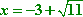is a zero of x4 + 6x3  7x2  30x + 10, fully solve the
equation
x4 + 6x3  7x2  30x + 10 = 0.

Since they have given me one of the zeroes, I'll use synthetic division to divide it out: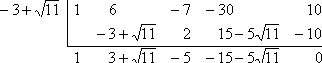(You will probably want to use scratch paper for the computations required when manipulating the radical root.) Copyright © Elizabeth Stapel 2002-2011 All Rights Reserved

Since you only get these square-root answers by using the Quadratic Formula, and since the square-root part of the Formula is preceded by a "plus-minus" sign, then these square-root answers must always come in pairs. Thus, if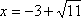is a root, then so also must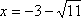be a root. So my next step is to divide by: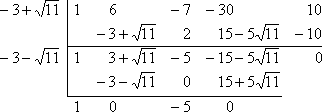I had started with a fourth-power polynomial. After the first division, I was left with a cubic (with very nasty coefficients!). After the second division, I'm now down to a quadratic (x2 + 0x  5, or just x2  5), which I know how to solve: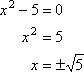Then the full solution is: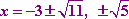If you have studied complex numbers, then you may see a problem of the following type.

• Given that 2  i is a zero of x5  6x4 + 11x3  x2  14x + 5, fully solve the
equation
x5  6x4 + 11x3  x2  14x + 5 = 0.

They have given us a zero, so I'll use synthetic division and divide out 2  i: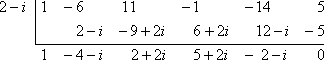(You will probably want to use scratch paper for the computations required when doing complex division.)

Recall that, to arrive at a zero of 2  i, they must have used the Quadratic Formula, which always spits out complex answers in pairs. That is, you get the imaginary part (the part with the "i") from having a negative inside the "plus or minus square-root of" part of the Formula. This means that, since 2  i is a zero, then 2 + i must also be a zero.  So I'll divide by 2 + i: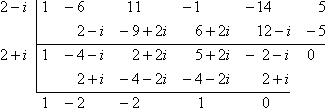This leaves me with a cubic, so I'll need to find another zero on my own. (That is, I can't apply the Quadratic Formula yet.) I can use the Rational Roots Test to help find potential zeroes, and a quick graph of x3  2x2  2x + 1 can help. I will try x = 1: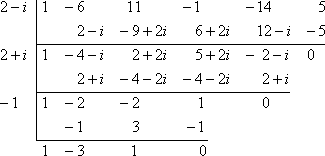Now I'm down to a quadratic (x2  3x + 1, which happens not to factor), so I'll apply the Quadratic Formula to get: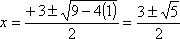Then all the zeroes of x5  6x4 + 11x3  x2  14x + 5 are given by: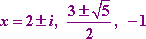The examples above repeatedly refer to the relationship between factors and zeroes. In other lessons (for instance, on solving polynomials), these concepts will be made more explicit. For now, be aware that checking a graph (if you have a graphing calculator) can be very helpful for finding test zeroes for doing synthetic division, and that a zero remainder after synthetic division by x = a means that x  a is a factor of the polynomial. If you do not have access to a graphing calculator for helping you find the right zeroes to try, there are some tricks you can use.

For an explanation of why synthetic division works (and for information on a variant method which will work for non-linear divisors), look at the Adobe Acrobat file titled "How Synthetic Division Works, or, The Madness Behind the Method", written by Walter Kehowski of Glendale Community College in Arizona.

<< Previous  Top  |  1 | 2 | 3 | 4  |  Return to Index

 Cite this article as: Stapel, Elizabeth. "Synthetic Division & Factoring." Purplemath. Available from     https://www.purplemath.com/modules/synthdiv4.htm. Accessed [Date] [Month] 2016

Study Skills Survey

Tutoring from Purplemath
Find a local math tutor

 Copyright © 2021  Elizabeth Stapel   |   About   |   Terms of Use   |   Linking   |   Site Licensing Contact Us